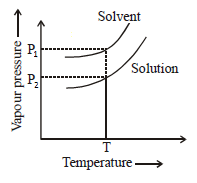You’ve reached the end of your free Videos limit.
#11 | Depression in Freezing Point
(Chemistry) > Solutions
Related Practice Questions :

50 g of antifreeze (ethylene glycol) is added to 200g water. What amount of ice will separate out at
-9.3°C. (Kf = 1.86 K Kg mol-1) :-

(1) 42 mg

(2) 42 g

(3) 38.71 g

(4) 38.71 mg

Complete Question Bank + Test Series
Complete Question Bank

Difficulty Level:

40 g NaOH (i=2) is dissolved in 1L volatile solvent vapour pressure  of this solution becomes equal to its solid phase at 300K. What is the freezing point of pure solvent.

(Density = 1g/mL) (kf=1.8 k kgmol-1)

1. 296.4 K

2. 303.6 K

3. 297.5 K

4. 302.4 K

Complete Question Bank + Test Series
Complete Question Bank

Difficulty Level:

When 36 g of a solute having the empirical formula CH2O is dissolved in 1.2 kg of water, the solution freezes at
-0.93°C. What is the molecular formula of solute (K= 1.86 kg K mol-1)

(1) C2H4O

(2) C2H2O2
(3) C2H4O3

(4) C2H4O2

Complete Question Bank + Test Series
Complete Question Bank

Difficulty Level:

P1 and P2 are vapour pressure of solvent and solution at temperature T. The solution is prepared by dissolving non-volatile solute in the same solvent. P1=760 torr, P2 = 684mm Hg, Mole fraction of solvent in the solution will be :-(1) 0.1

(2) 0.2

(3) 0.9

(4) Can't be predicted

Complete Question Bank + Test Series
Complete Question Bank

Difficulty Level:

A non-volatile solute is dissolved in a water. If its degree of association is 50%. What will be its freezing point when boiling point is 100.52$°\mathrm{C}$. [Kb=0.52 K kg mol-1, Kf=1.86K kg mol-1]

(1) -0.52 K

(2) -0.52$°\mathrm{C}$

(3) -1.86$°\mathrm{C}$

(4) 275.01 K